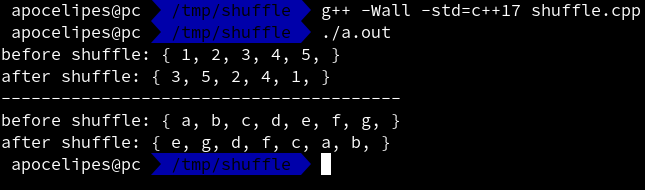# c++随机排序容器中的元素

template< class RandomIt, class URBG >
void shuffle( RandomIt first, RandomIt last, URBG&& g );


shuffle调用后目标容器内的元素排列顺序会被随机打乱，我们看个例子。

// 帮助函数，打印vector的内容
template <typename T>
std::ostream &operator<<(std::ostream &os, const std::vector<T> &v)
{
os << "{ ";
for (const auto &i: v) {
os << i << ", ";
}
os << "}";

return os;
}

// 帮助函数，生成一个符合UniformRandomBitGenerator要求的随机数生成器；
// std::random_device虽然符合要求但是只适合于生成seed及安全要求较高的场合，因为速度可能很慢。
// 所以我们选择std::mt19937算法，你可以自己选择其他合适的算法
auto get_URBG()
{
std::random_device rd;
// 使用random_device生成seed
std::mt19937 g(rd());

return g;
}


// 随机排序容器内元素，打印随机排序前和随机排序后的容器内容
template <typename T>
void shuffle_container(std::vector<T> &container)
{
std::cout << "before shuffle: " << container << std::endl;
std::shuffle(container.begin(), container.end(), get_URBG());
std::cout << "after shuffle: " << container << std::endl;
}

int main()
{
std::vector<int> ivec{1,2,3,4,5};
shuffle_container(ivec);

// 分割线
std::cout << std::string(40, '-') << std::endl;

std::vector<std::string> svec{"a", "b", "c", "d", "e", "f", "g"};
shuffle_container(svec);
}posted @ 2019-02-04 01:41  apocelipes  阅读(2981)  评论(0编辑  收藏  举报